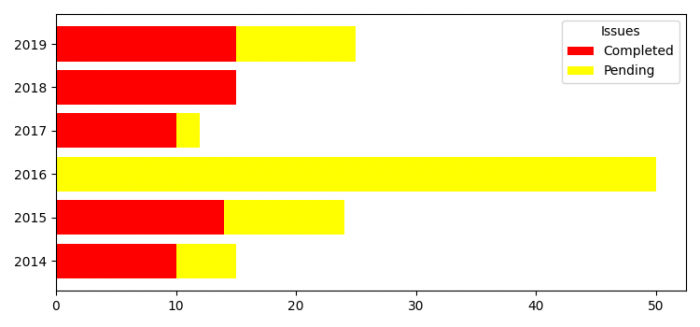# Horizontal stacked bar chart in Matplotlib

To plot stacked bar chart in Matplotlib, we can use barh() methods

## Steps

• Set the figure size and adjust the padding between and around the subplots.
• Create a list of years, issues_addressed and issues_pending, in accordance with years.
• Plot horizontal bars with years and issues_addressed data.
• To make stacked horizontal bars, use barh() method with years, issues_pending and issues_addressed data
• Place the legend on the plot.
• To display the figure, use show() method.

## Example

from matplotlib import pyplot as plt
plt.rcParams["figure.figsize"] = [7.50, 3.50]
plt.rcParams["figure.autolayout"] = True

year = [2014, 2015, 2016, 2017, 2018, 2019]
issues_addressed = [10, 14, 0, 10, 15, 15]
issues_pending = [5, 10, 50, 2, 0, 10]

plt.show()